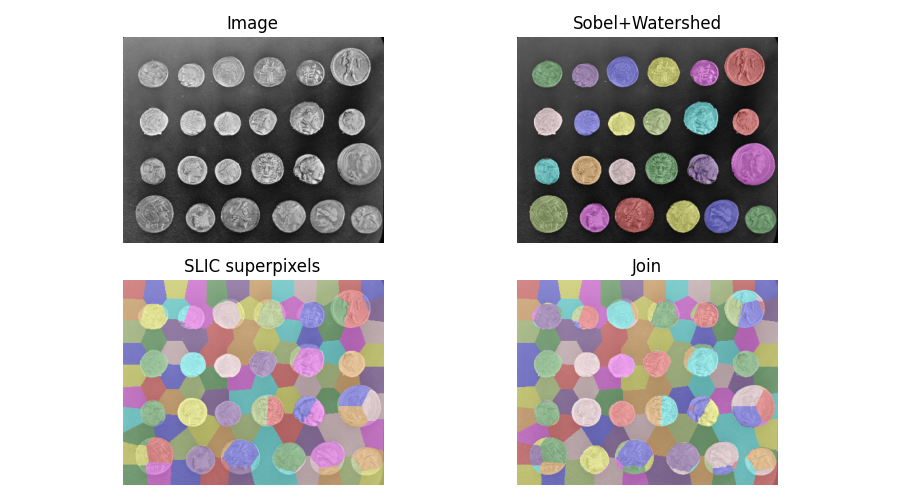# Find the intersection of two segmentations¶

When segmenting an image, you may want to combine multiple alternative segmentations. The `skimage.segmentation.join_segmentations()` function computes the join of two segmentations, in which a pixel is placed in the same segment if and only if it is in the same segment in both segmentations.```import numpy as np
import matplotlib.pyplot as plt

from skimage.filters import sobel
from skimage.measure import label
from skimage.segmentation import slic, join_segmentations, watershed
from skimage.color import label2rgb
from skimage import data

coins = data.coins()

# Make segmentation using edge-detection and watershed.
edges = sobel(coins)

# Identify some background and foreground pixels from the intensity values.
# These pixels are used as seeds for watershed.
markers = np.zeros_like(coins)
foreground, background = 1, 2
markers[coins < 30.0] = background
markers[coins > 150.0] = foreground

ws = watershed(edges, markers)
seg1 = label(ws == foreground)

# Make segmentation using SLIC superpixels.
seg2 = slic(coins, n_segments=117, max_num_iter=160, sigma=1, compactness=0.75,
channel_axis=None, start_label=0)

# Combine the two.
segj = join_segmentations(seg1, seg2)

# Show the segmentations.
fig, axes = plt.subplots(ncols=2, nrows=2, figsize=(9, 5),
sharex=True, sharey=True)
ax = axes.ravel()
ax.imshow(coins, cmap='gray')
ax.set_title('Image')

color1 = label2rgb(seg1, image=coins, bg_label=0)
ax.imshow(color1)
ax.set_title('Sobel+Watershed')

color2 = label2rgb(seg2, image=coins, image_alpha=0.5, bg_label=-1)
ax.imshow(color2)
ax.set_title('SLIC superpixels')

color3 = label2rgb(segj, image=coins, image_alpha=0.5, bg_label=-1)
ax.imshow(color3)
ax.set_title('Join')

for a in ax:
a.axis('off')
fig.tight_layout()
plt.show()
```

Total running time of the script: ( 0 minutes 0.734 seconds)

Gallery generated by Sphinx-Gallery